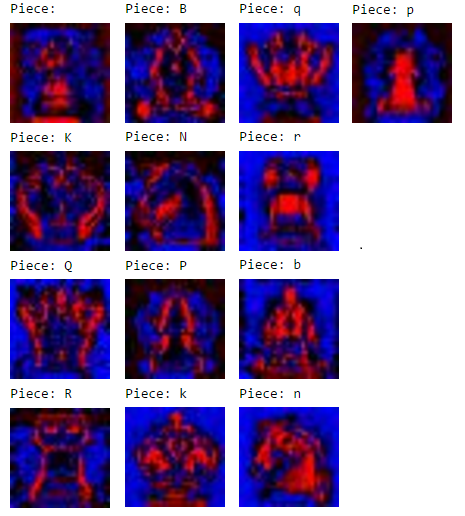### Learning TensorFlow #2 - Predicting chess pieces from images using a single-layer classifier

Let's train a tensorflow neural network to tell what piece is on a chess square. In the previous post discussed how to parse input images which contained a chessboard into 32x32 grayscale chess squares. Let's look again at our input and outputs.

Input
• 32x32 grayscale normalized image of a single chess tile containing a piece or emptyExample input tile, a black pawn
Output
• A label for which piece we think it is, there are 6 white pieces and 6 black pieces, and 1 more for an empty square, so 13 possible choices.

Let's define our output label as an integer value from 0-12, where 0 is an empty square, 1-6 is white King, Queen, Rook, Bishop, Knight Pawn, and then 7-12 are the same for black. A black pawn in this case would be 12 then. In a one-hot label vector, this would be [0, 0,0,0,0,0,0, 0,0,0,0,0,1], where the 12th index is 1 and the rest are zero.

How do we generate training data where we know the labels? One way is to take screenshots of the starting chessboard position, where we know exactly where all the pieces are supposed to be. I went ahead and took around 14 screenshots of lichess.org starting positions for a few themes and piece sets. This gave us around 900 tiles (64 tiles per image) to train with. It was a bit limited but it's enough for a basic classifier.

## Building a Model

We followed the MNIST tensorflow tutorial almost verbatim, building a simple classifier, you can follow along directly from the IPython notebook as well, or continue with the summary below.

```x = tf.placeholder(tf.float32, [None, 32*32])
W = tf.Variable(tf.zeros([32*32, 13]))
b = tf.Variable(tf.zeros())

y = tf.nn.softmax(tf.matmul(x, W) + b)
y_ = tf.placeholder(tf.float32, [None, 13])

cross_entropy = -tf.reduce_sum(y_*tf.log(y))

```

Our inputs are 32x32 normalized grayscale images, which we unroll into a 1024 length vector of floats. Our weight matrix is a fully connected matrix multiply of a 1024 x 13 array, 13 being the length of the output vector of probabilities associated with each piece. We then define a cross_entropy variable which is based on the difference between our expected label vector and our predicted vector of probabilities. We use Gradient Descent to minimize this value, amazingly tensorflow simplifies this to a single line!

We can then train our model and test it's accuracy on a testing dataset we kept separate from teh training dataset:

```correct_prediction = tf.equal(tf.argmax(y,1), tf.argmax(y_,1))
accuracy = tf.reduce_mean(tf.cast(correct_prediction, "float"))
print "Accuracy: %g\n" % sess.run(accuracy, feed_dict={x: test_dataset.images, y_: test_dataset.labels})

> Accuracy: 0.996142
```

99.6% accuracy, that's pretty impressive, but less impressive when we realize we're only testing it against a testing dataset we collected, from the same sites we built our training dataset on. In the real world with images from other sites, this classifier has and will fail much more often.

Let's visualize our weights to get a sense of how the classifier is looking for each piece:Weights for each piece, red is negative, blue is positive
Awesome! We're able to see a concept of what the neural network is looking for in each of the pieces. We can wrap this together into a prediction suite, where we pass in an image url, we use CV to break up the screenshot into input tiles, the neural network predicts each piece for the 64 tiles, which we then compile into a single board layout in FEN notation.

```def getPrediction(img):
"""Run trained neural network on tiles generated from image"""

# Convert to grayscale numpy array
img_arr = np.asarray(img.convert("L"), dtype=np.float32)

# Use computer vision to get the tiles
tiles = tensorflow_chessbot.getTiles(img_arr)
if tiles is []:
print "Couldn't parse chessboard"
return ""

# Reshape into Nx1024 rows of input data, format used by neural network
validation_set = np.swapaxes(np.reshape(tiles, [32*32, 64]),0,1)

# Run neural network on data
guess_prob, guessed = sess.run([y, tf.argmax(y,1)], feed_dict={x: validation_set})

# Convert guess into FEN string
# guessed is tiles A1-H8 rank-order, so to make a FEN we just need to flip the files from 1-8 to 8-1
pieceNames = map(lambda k: '1' if k == 0 else hf.labelIndex2Name(k), guessed) # exchange ' ' for '1' for FEN
fen = '/'.join([''.join(pieceNames[i*8:(i+1)*8]) for i in reversed(range(8))])
return fen

def makePrediction(image_url):
"""Given image url to a chessboard image, return a visualization of FEN and link to a lichess analysis"""
# Load image from url and display

print "Image on which to make prediction: %s" % image_url

# Make prediction
fen = getPrediction(img)
display(Markdown("Prediction: [Lichess analysis](http://www.lichess.org/analysis/%s)" % fen))
display(Image(url='http://www.fen-to-image.com/image/%s' % fen))
print "FEN: %s" % fen
```

And let's try it out! All the boilerplate is done, the model is trained, it's time. I chose the first post I saw on /r/chess with a chessboard and an image url:First prediction, success!
A great success on the first prediction. It was able to handle the highlighting on the pawn movement from G2 to F3 also. The reason it worked so well here is this image url is to a screenshot of a lichess image, a piece set and theme we have trained for, so it's reasonable to expect success here. Fantastic, a perfect match!

Now just for fun, let's try an image that is from a chessboard we've never seen before! Here's another on reddit:Attempt on image from unknown chess site, failure on black and white pawns to bishops
Hah, it thought all the pawns were bishops. This is a good example of where our training dataset does not encompass enough variations in piece themes, though it was nice to see that the network was able to predict several other pieces and all the blank squares successfully, very nice.

In the next post I discuss improving our neural network by building a larger dataset, as well as making the jump to a larger convolutional neural network, which is most definitely overkill, but quite fun.

For a sneak peek of the final product, check out the repository on Github